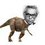# 1 equation , 2 variables.Solvable?

$\large a^3+b^3+3ab=1$

If $$a,b \in R$$ , Find value of $$a+b$$

Nice solutions are always welcome!Note by Nihar Mahajan
3 years, 11 months ago

MarkdownAppears as
*italics* or _italics_ italics
**bold** or __bold__ bold
- bulleted- list
• bulleted
• list
1. numbered2. list
1. numbered
2. list
Note: you must add a full line of space before and after lists for them to show up correctly
paragraph 1paragraph 2

paragraph 1

paragraph 2

[example link](https://brilliant.org)example link
> This is a quote
This is a quote
    # I indented these lines
# 4 spaces, and now they show
# up as a code block.

print "hello world"
# I indented these lines
# 4 spaces, and now they show
# up as a code block.

print "hello world"
MathAppears as
Remember to wrap math in $$...$$ or $...$ to ensure proper formatting.
2 \times 3 $$2 \times 3$$
2^{34} $$2^{34}$$
a_{i-1} $$a_{i-1}$$
\frac{2}{3} $$\frac{2}{3}$$
\sqrt{2} $$\sqrt{2}$$
\sum_{i=1}^3 $$\sum_{i=1}^3$$
\sin \theta $$\sin \theta$$
\boxed{123} $$\boxed{123}$$

Sort by:

a+b can be 1 also

- 3 years, 11 months ago

Yeah it can be $$1$$. So?

- 3 years, 11 months ago

LOL xD

- 3 years, 11 months ago

The answer is $$\space \boxed{a+b=1}$$.

I plotted $$f(a) = a^3+b^3+3ab-1$$ for $$b = -10, -7.5, 0, 7.5, 10$$, thinking that there will be infinite solutions. But I discovered that the roots to $$f(a)$$ are respectively (a=11,8.5,1,-6.5,-9) making $$\boxed{a+b = 1}$$. See the graph here.- 3 years, 11 months ago

There is one special case when $$a+b=-2$$.

- 3 years, 11 months ago

Sir , note that $$a+b=-2$$ is also a solution. See my and Krishna's solution. Thanks!

- 3 years, 11 months ago

$$\displaystyle a^3 + b^3 + c^3 -3abc = \dfrac{1}{2} (a+b+c)( (a-b)^2 + (b-c)^2 + (c-a)^2)$$

Now substitute $$c = -1$$ and then L.H.S equals 0

$$\displaystyle 0 = (a+b-1)( (a-b)^2 + (a+1)^2 + (b+1)^2)$$

From here

$$a+b = 1$$ from the first and

$$a+b = -2$$ from second (easily solvable)

EDIT :- the second equation has only one solution when $$a =-1$$.

- 3 years, 11 months ago

That identity is very useful it is just a result derived from another identity.

- 3 years, 10 months ago

Nice , that crucial substitution really helped!

- 3 years, 11 months ago

So , here is my trial:

$a^3+b^3+3ab-1=0 \\ (a+b)^3-3ab(a+b)+3ab-1=0 \\ (a+b)^3 - 3ab(a+b-1) -1=0 \\ (a+b)^3 - 1^3 = 3ab(a+b-1) \\ (a+b-1)(a^2+b^2+2ab+a+b+1)= 3ab(a+b-1)$

Let $$a+b-1 \neq 0$$

$\Rightarrow a^2+b^2+2ab+a+b+1= 3ab \\ a^2+b^2-ab+a+b+1=0 \\ a^2+b^2-(a-1)(b-1)=0 \\ a^2+b^2=(a-1)(b-1) \\ (a+b)^2 - 2ab = (a-1)(b-1) \\ (a+b+\sqrt{2ab})(a+b-\sqrt{2ab})=(a-1)(b-1) \\ \Rightarrow b+\sqrt{2ab} = -1 \quad a-\sqrt{2ab}=-1 \\ \Rightarrow a+b=-2 \quad a+b=1$

Is it right?

- 3 years, 11 months ago

Please explain the second last step

- 3 years, 11 months ago

$(a+b+\sqrt{2ab})(a+b-\sqrt{2ab})=(a-1)(b-1) \\ \Rightarrow (a+(b+\sqrt{2ab}))(b+(a-\sqrt{2ab}))=(a-1)(b-1)$

If you compare the factors of $$L.H.S$$ and $$R.H.S$$ , correspondingly you will get:

$\Rightarrow b+\sqrt{2ab} = -1 \quad a-\sqrt{2ab}=-1$

- 3 years, 11 months ago

Hey ,in the 5th step ,from where has -1 arrived in the second bracket I mean when you used the formula $$a^{3} - b^{3}$$ the correct equation should have been this $$(a^{2} + b^{2} + 2ab +1 +a+b$$)

- 3 years, 11 months ago

Sorry , it was a typing mistake. Thanks for correcting me.

- 3 years, 11 months ago

Note @Mehul Arora @Vaibhav Prasad , $$a,b$$ are real numbers and not just integers. Cheers!

- 3 years, 11 months ago

yup, it's right

- 3 years, 11 months ago

Do like and reshare this for others (not for my sake). Thanks!

- 3 years, 11 months ago

Let a+b=x Now If, x=1, Then the Equation becomes a+b ^ 3 Thena+b=1 :D

P.S. I'm just too lazy. Idk What I am typing right now.

- 3 years, 11 months ago

That's right.

- 3 years, 11 months ago

I know.

- 3 years, 11 months ago

But that's incomplete. -_-

- 3 years, 11 months ago

Somewhat. But I laid the basis, didn't I ? :P

- 3 years, 11 months ago

Yeah you have a nice different idea. I am trying a different approach... xD

- 3 years, 11 months ago

Okay! So Cheers! xD

- 3 years, 11 months ago

See my bash , no need to compute $$a,b$$ :)

- 3 years, 11 months ago

How about $$a=-1$$ and $$b=-1$$

- 3 years, 11 months ago

See my bash , no need to compute $$a,b$$ :)

- 3 years, 11 months ago

I am not asking for the answer. I am asking for an elegant accurate solution with proof. -_-

- 3 years, 11 months ago

1 :- The answer

- 3 years, 11 months ago

I am not asking for the answer. I am asking for an elegant accurate solution with proof. -_-

- 3 years, 11 months ago

- 3 years, 11 months ago

What do you need help with? You solved the problem didn't you? :)

- 3 years, 11 months ago

Actually , I got it just after I posted this note. Since you have nice methods and approaches , I mentioned you.

- 3 years, 11 months ago